2022 NL Cy Young Odds
+320
3.2 to 1Corbin Burnes
16.6% implied probability

+700
7 to 1Carlos Rodon
8.7% implied probability

+1000
10 to 1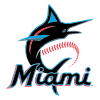Pablo Lopez
6.3% implied probability

+1100
11 to 1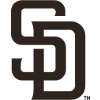Joe Musgrove
5.8% implied probability

+1100
11 to 1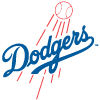Walker Buehler
5.8% implied probability

+1500
15 to 1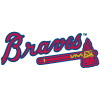Max Fried
4.4% implied probability

+1500
15 to 1Zack Wheeler
4.4% implied probability

+1800
18 to 1Zac Gallen
3.7% implied probability

+2000
20 to 1Sandy Alcantara
3.3% implied probability

+2800
28 to 1Brandon Woodruff
2.4% implied probability

+2800
28 to 1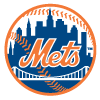Max Scherzer
2.4% implied probability

+3000
30 to 1Aaron Nola
2.3% implied probability

+3500
35 to 1Kyle Wright
1.9% implied probability

+3500
35 to 1Clayton Kershaw
1.9% implied probability

+3500
35 to 1Chris Bassitt
1.9% implied probability

+4000
40 to 1Julio Urias
1.7% implied probability

+4000
40 to 1Eric Lauer
1.7% implied probability

+4000
40 to 1Logan Webb
1.7% implied probability

+5000
50 to 1Yu Darvish
1.4% implied probability

+5000
50 to 1Josh Hader
1.4% implied probability

+5000
50 to 1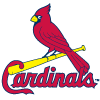Miles Mikolas
1.4% implied probability

+5000
50 to 1Sean Manaea
1.4% implied probability

+6000
60 to 1Freddy Peralta
1.1% implied probability

+8000
80 to 1Jacob deGrom
0.9% implied probability

+8000
80 to 1Carlos Carrasco
0.9% implied probability

+9000
90 to 1Tylor Megill
0.8% implied probability

+9000
90 to 1Kyle Gibson
0.8% implied probability

+10000
100 to 1Alex Cobb
0.7% implied probability

+10000
100 to 1Ranger Suarez
0.7% implied probability

+10000
100 to 1Merrill Kelly
0.7% implied probability

+10000
100 to 1Adam Wainwright
0.7% implied probability

+10000
100 to 1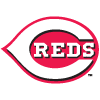Luis Castillo
0.7% implied probability

+10000
100 to 1Ian Anderson
0.7% implied probability

+10000
100 to 1German Marquez
0.7% implied probability

+10000
100 to 1Charlie Morton
0.7% implied probability

+10000
100 to 1Anthony DeSclafani
0.7% implied probability

+10000
100 to 1Andrew Heaney
0.7% implied probability

+10000
100 to 1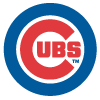Marcus Stroman
0.7% implied probability

+10000
100 to 1Adrian Houser
0.7% implied probability

+10000
100 to 1Blake Snell
0.7% implied probability

+10000
100 to 1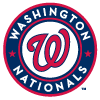Patrick Corbin
0.7% implied probability

+10000
100 to 1Steven Matz
0.7% implied probability

+10000
100 to 1Stephen Strasburg
0.7% implied probability

+10000
100 to 1Wade Miley
0.7% implied probability

+10000
100 to 1Madison Bumgarner
0.7% implied probability

+10000
100 to 1Jack Flaherty
0.7% implied probability